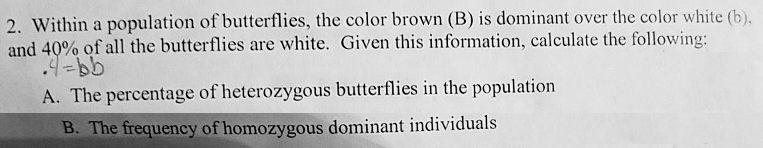# Problem: Within a population of butterflies, the color brown (B) is dominant over the color white (b), and 40% of all the butterflies are white. Given this information, calculate the following:A. The percentage of heterozygous butterflies in the population.B. The frequency of homozygous dominant individuals.

###### FREE Expert Solution

Assuming the population follows hardy Weinberg equilibrium, the percentage of white butterflies, 40% or 0.4, is equivalent to q2. Thus, the value of q is the square root of 0.4, which is 0.632.###### Problem Details

Within a population of butterflies, the color brown (B) is dominant over the color white (b), and 40% of all the butterflies are white. Given this information, calculate the following:

A. The percentage of heterozygous butterflies in the population.

B. The frequency of homozygous dominant individuals.